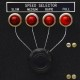Double-rounding in floating-point literal conversion

XMLWordPrintable

Details

• Type:Bug
• Status: Open
• Priority:Medium
• Resolution: Unresolved
• Component/s:
• Labels:
None

Description

Floating-point literals are currently converted first to `_MaxBuiltinFloatType`, and then to the actual type. This rounds twice, which necessarily brings up the possibility of double rounding errors. Here's a concrete example of this in action on x86, where we round first to `Float80`:

1> let x = 1.000000000000000111076512571139929264063539449125528335571

x: Double = 1

`1` is incorrect; the "correct" (single-rounding) double result is `1.0000000000000002` (the literal is just barely larger than the halfway point between the two values, but the initial rounding to `Float80` rounds exactly to the halfway point, so the subsequent rounding rounds down to `1`).

How do we fix this? The usual approach is to make the first rounding happen in a special rounding mode, "round to odd", which rounds any non-exact value to the representable number whose least significant bit is set. This solves the problem for any type that's more than one bit smaller than the intermediate type, but would result in us getting the wrong result if they're the same (then we should be rounding to nearest instead).

We could solve this for concrete built-in types (by far the more important thing to fix) by rounding first to an intermediate type with, say, 32b exponent and 128b significand under the round-to-odd rule (this would also let us implement `Float128` in the future without further changes). This would not solve the problem in general for arbitrary user-defined binary floating-point types, since we don't know how large they may be; we should really be providing them with something like a hexadecimal digit sequence in the longer term.

People

Assignee:Unassigned
Reporter:Stephen Canon